# How to Count the Number of Sheets in a Workbook

This post will guide you how to count the number of sheets in a workbook in Excel. How do I count the number of worksheets in a workbook with VBA Macro in Excel.

## Count the Number of Sheets with Define Name

If you want to count the number of worksheets in a given workbook in Excel, you can use the Defined Name and a Formula to achieve it. Just do the following steps:

#1 go to Formula tab, click Define Name command under Defined Names group, and the New Name dialog will open.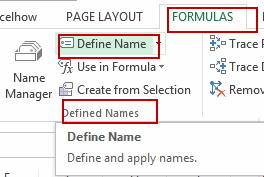#2 type one defined name in the Name text box, such as: countWorksheets, and then type the formula =GET.WORKBOOK(1)&T(NOW()) into the text box of Refers to. Click Ok button.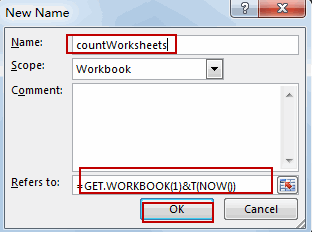#3 Type the following formula based on the COUNTA function and the INDEX function to get the number of worksheets in the current workbook. And press Enter key in your keyboard, you will get the number of worksheets in your workbook.

`=COUNTA(INDEX(CountWorksheets,0))`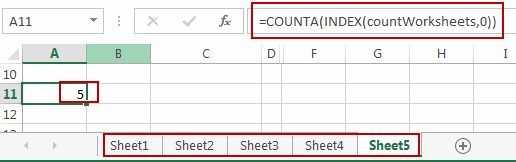## Count the Number of Sheets with VBA Macro

You can also use an Excel VBA Macro to get the number of worksheets in the current workbook. Just do the following steps:

#1 open your excel workbook and then click on “Visual Basic” command under DEVELOPER Tab, or just press “ALT+F11” shortcut.#2 then the “Visual Basic Editor” window will appear.

#3 click “Insert” ->”Module” to create a new module.#4 paste the below VBA code into the code window. Then clicking “Save” button.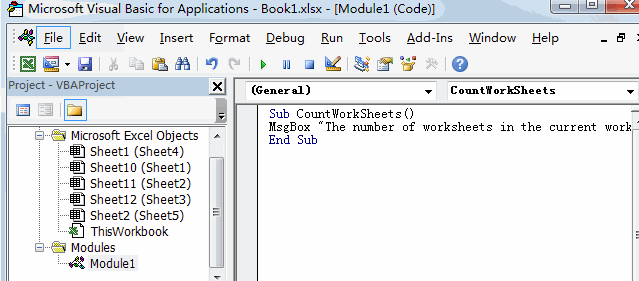```Sub CountWorkSheets()
MsgBox "The number of worksheets in the current workbook is : " & Application.Sheets.Count
End Sub```

#5 back to the current worksheet, then run the above excel macro. Click Run button.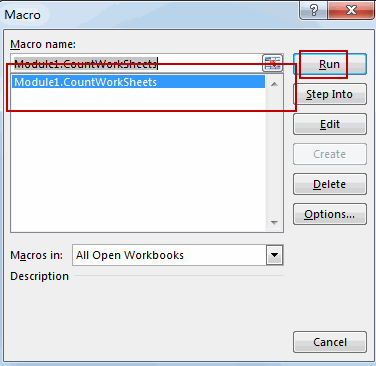#6 let’s see the result: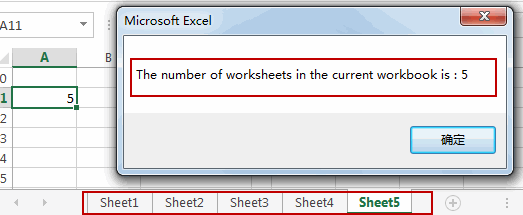Video: Count the Number of Sheets

Related Posts

VBA Macro For VLOOKUP From Another Sheet

In the previous post, you should know that how to fix or remove the #N/A error when using VLOOKUP formula to lookup value from another sheet. And this post will show you how to use VBA code to vlookup data ...

How To Insert Comments in Protected Worksheet in Excel

This post will show you how to allow comments in a protected worksheet in Excel. You can easily to insert comments into cells in a normal worksheet in Excel, but if want to insert a comment in a worksheet that ...

How To Convert Text to Upper Cases(Using VBA) in Excel

This post will show you how to switch from lower case to upper case in Excel. and I am going to show you two different ways of converting text to upper cases using formula or VBA macro in Excel 2013,Excel ...

How To Hide Every Other Row in Excel (Using VBA)

This post will show you how to hide alternate rows or columns in Excel or how to hide every third, fourth, fifth row or column in Excel. If you want to hide every other row in your current worksheet, how ...

How to Disable the Save As Prompt in Excel

This post will show you how to use a VBA Macro to save an Excel file and overwrite any existing file without a prompt so that you are going to get the little window that says file already exists do ...

How to Count Cells that Contain even or odd numbers in Excel

This post will guide you how to count the number of cells that contain odd or even numbers within a range of cells using a formula in Excel 2013/2016.How do I count cells that contain odd numbers through the use ...

How to Count Cells that Contain negative Numbers in Excel

This post will guide you how to count the number of cells that contain negative numbers within a range of cells using a formula in Excel 2013/2016.You can count the number of negative numbers in your data using easy functions ...

How to Count Cells Are Not Blank or Empty in Excel

This post will guide you how to count cells that are not blank or empty in a given range cells using a formula in Excel 2013/2016.How do I count the number of cells that are not blank in a particular ...

How to Count Cells Less Than a Specific Value in Excel

This post will guide you how to count the number of cells less than a particular numeric value in a given range cells using a formula in Excel 2013/2016. How do I count cells that are less than a specific ...

How to Count Cells Greater Than a Specific Value in Excel

This post will guide you how to count the number of cells greater than a particular numeric value in a given range cells using a formula in Excel 2013/2016. How do I count cells that are greater than a specific ...

Sidebar